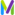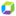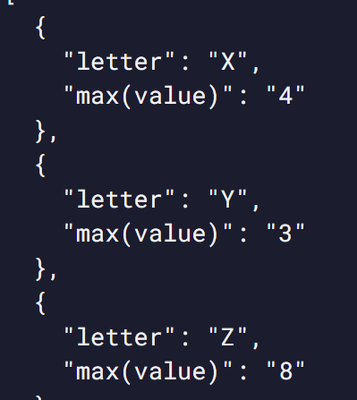cancel
Showing results for
Show  only  | Search instead for
Did you mean:DynaMight Champion

Hello,

Does DQL also do “bucket” aggregations?

Kr Henk

example:

x = 4

y =3

z =8

y = 2

x = 2

query would be, as an example: give me the highest number per letter,

x = 4

y = 3

z = 8

Or a dynatrace example, give me the top cpu load per host.

2 REPLIES 2Dynatrace Champion

Hello,

i hope i understood your dataset, but here is a query you could copy paste and see whether it meets your expectations:

``````data record(letter="X", value=4), record(letter="Y", value=3), record(letter="Z", value=8),
record(letter="X", value=3), record(letter="Y", value=2), record(letter="Z", value=0)
| summarize max(value), by:letter``````DynaMight ChampionHello Peter,

Thanks for your reply, much easier than the competitor (-; I was making it much to difficult, you made my day, thanks!

KR HenkFeatured Posts